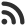Feed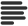Articles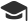Tutorials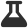Lab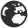CompaniesLeaderboard
 HS Intern at Microsoft March 31, 2020, 7:31 p.m. ⋅ 277 views

# Maximum sum such that no two elements are adjacent

We are given an array of positive integres, and we need to find the sum of any subsequence in which any none of the any 2  element is adjacet element in the given array.

In other words, we need to come up with a sub sequence of the given array, whose sum is highest and none of 2 elements are adjacent in given array.

Let's take an example.

Given array

``1 2 3 4 5``

Here 1 and 2 are adjecent, similarly, 2 and 3 are adjacent.

So, our answer would be 1 + 3 + 5 = 8. See we are not taking any element which are adjacent in array.

Another subsequnce with no adjacent elements would have been, 2, 4 but its total sum would be lesser so, that's not required answer.

I hope you have understood the problem clearly. Now, let's try to build an algorithm for it.

Solution

We'll loop over the array keeping 2 sums. We'll call them inclusive and exclusive. Why did we choose this name ?

Inclusive - Max sum including the previous element

Exlusive - Max sum excluding the previous element.

So, Inclusive can be given by, Exclusive + current element

and, Exclusive will be max(Inclusive, Exclusive)

Implementation

``````#include<bits/stdc++.h>
using namespace std;
int FindMaxSum(int arr[],int n)
{
int incl=arr; //we are taking this as arr because we are including arr value in sum
int excl=0;  //since we have no other after we exclude arr so thats why we are taking excl =0
for(int i=1;i<n;i++)
{
int temp=incl;
incl=max(temp,arr[i]+excl); // suppose at any point of time we are at arr so now temp will contain
// the value of arr since we can not use adjacent value so for that we are
// taking maximum of previous value of arr i.e arr and in this case it is
// temp  and if we are including arr value then we can use excl value that
// is value before arr in this case it is 0 because if we add that then it is not
excl=temp;                  // excl will go over all value starting from arr to arr[n-2]
}
return incl;
}
int main()
{
int arr[] = {1, 2, 3};
int n = sizeof(arr) / sizeof(arr);
cout<<FindMaxSum(arr, n);
return 0;
}``````
``4``

Events

Nov. 28, 2018, 5:30 p.m.

Python from zero to hero

place Delhi

Aug. 13, 2018, 5:30 p.m.

Python from zero to hero

place Bangalore ( HackersFriend office BTM Layout)